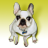# Field Calculator Subtract Value From Previous Value

4618
8
03-29-2013 06:17 AMNew Contributor II
Hello,

I have a field in my attribute table representing pipe lengths.  In an empty field (Distance) I would like to subtract each pipe length from the previous value, thereby calculating the distance.  Also, in the field calculator, I need to be able to sort the Pipe_Length field in ascending order before doing the calculation.  For example:

Pipe_Length.........Distance
0........................0
1615...................1615
4494...................2879
4630...................136

I have ArcGIS 9.3 and this MUST be done in the field calculator.  I have no access to ArcGIS 10 nor can I download an evaluation copy of it.  It must be done using 9.3 in the field calculator.  The sort ascending must also be done in the field calculator before doing the calculation.  Unfortunately I am not able to divert from 9.3 or the field calculator.  The result must be exactly how it shows in the above example.  My VB logic skills are pretty weak so that it why I am posting here!  Thank you for your help!
Tags (3)
1 Solution

Accepted SolutionsbyMVP Esteemed Contributor
ERROR 000539: Error running expression: CalcDiff(r"C:\Export_Output.dbf", "PIPELENGTH", "DISTANCE") <type 'exceptions.TypeError'>: 'geoprocessing cursor object' object is not iterable
Failed to execute (CalculateValue).

Sorry about that -- I forgot to use 9.3 syntax for cursors. Need to go look at the 9.3 help...

`import arcgisscripting gp = arcgisscripting.create(9.3) def CalcDiff(tbl,valField,diffField):   procFields = valField + ";" + diffField   sortField = valField   Rows = gp.UpdateCursor(tbl, "", "", procFields, sortField)   lastVal = 0   Row = Rows.next()   while Row:     val = float(Row.getValue(valField))     # calc the difference between the last value and this one     diff =  val - lastVal     Row.setValue(diffField,diff)     Rows.updateRow(Row)     lastVal = val     Row = Rows.next()   del Row, Rows   return tbl`
8 RepliesbyMVP Esteemed Contributor
The sort ascending must also be done in the field calculator before doing the calculation.

Sorry, Stuart, I have bad news for you. The Calculate Field  code block is executed one row at a time - so you are dependent on the sort order of the input table. (The Sort tool was (finally) introduced at 10.0 -- I have wanted it since 8.0 was released!)

Seems to me the best approach to this would be to do this in Python with a sorted update cursor instead of Calculate Field. You could create a script tool, but I bet you can get by using the Calculate Value tool in Model Builder:

Expression: CalcDiff(r"pipetable.dbf", "PIPE_LENGTH", "DISTANCE")

Code Block:

```import arcgisscripting
gp = arcgisscripting.create(9.3)
def CalcDiff(tbl,valField,diffField):
procFields = valField + ";" + diffField
sortField = valField
Rows = gp.UpdateCursor(tbl, "", "", procFields, sortField)
lastVal = 0
for Row in Rows:
val = float(Row.getValue(valField))
# calc the difference between the last value and this one
diff =  val - lastVal
Row.setValue(diffField,diff)
Rows.updateRow(Row)
lastVal = val
del Row, Rows
return tbl
```

Data Type: TableNew Contributor II
Curt,

Thank you for responding!  I am very interested in your recommendation of the Calculate Value tool.  I tried running the tool using the expression and code you provided, however, I am having some issues.  I am getting the following error:

ERROR 000539: Error running expression: CalcDiff(r"Export_Output.dbf", "PIPELENGTH", "DISTANCE") <type 'exceptions.RuntimeError'>: ERROR 999999: Error executing function.
A locator with this name does not exist.
Failed to execute (CalculateValue).

Do I need to change anything in the Code Block to reflect my specific data?  Does the table need to be in the ArcMAP TOC?  My Python skills are similar to my VBA skills.....weak!  Thanks for your help on this.byMVP Esteemed Contributor
Stuart, I don't see the problem, two things to check:

1. Make sure your dbf table listed there is in the current workspace or a fully specified path, for example:

CalcDiff(r"C:\workspace\pipetable.dbf", "PIPE_LENGTH", "DISTANCE")

if this is a model element named Input table you can do this:

CalcDiff(r"%Input table%", "PIPE_LENGTH", "DISTANCE")

2. Function and variable names case-sensitive in Python, so make sure you have the caps right.New Contributor II
Curt,

I tried using the fully specified path of the table and I got this error:

ERROR 000539: Error running expression: CalcDiff(r"C:\Export_Output.dbf", "PIPELENGTH", "DISTANCE") <type 'exceptions.TypeError'>: 'geoprocessing cursor object' object is not iterable
Failed to execute (CalculateValue).

Any ideas?  Thanks.byMVP Esteemed Contributor
ERROR 000539: Error running expression: CalcDiff(r"C:\Export_Output.dbf", "PIPELENGTH", "DISTANCE") <type 'exceptions.TypeError'>: 'geoprocessing cursor object' object is not iterable
Failed to execute (CalculateValue).

Sorry about that -- I forgot to use 9.3 syntax for cursors. Need to go look at the 9.3 help...

`import arcgisscripting gp = arcgisscripting.create(9.3) def CalcDiff(tbl,valField,diffField):   procFields = valField + ";" + diffField   sortField = valField   Rows = gp.UpdateCursor(tbl, "", "", procFields, sortField)   lastVal = 0   Row = Rows.next()   while Row:     val = float(Row.getValue(valField))     # calc the difference between the last value and this one     diff =  val - lastVal     Row.setValue(diffField,diff)     Rows.updateRow(Row)     lastVal = val     Row = Rows.next()   del Row, Rows   return tbl`New Contributor II
That worked!!  Thank you very much for your help on this!!New Contributor II
Curt,

You're probably going to think I'm crazy but now I need to do this in 10.1.  What is the syntax I should be using?  Thanks.byMVP Esteemed Contributor
What is the syntax I should be using?  Thanks.

Fortunately, scripts written for 9.3 (using arcgisscripting) should work fine at 10.x. This includes scripts dropped into the Calculate Value tool.

Here's it is set up for arcpy, anyway, just so you can see the slight difference.

```import arcpy
def CalcDiff(tbl,valField,diffField):
procFields = valField + ";" + diffField
sortField = valField
Rows = gp.UpdateCursor(tbl, "", "", procFields, sortField)
lastVal = 0
while Row:
val = float(Row.getValue(valField))
# calc the difference between the last value and this one
diff =  val - lastVal
Row.setValue(diffField,diff)
Rows.updateRow(Row)
lastVal = val
del Row, Rows
return tbl
```

ArcGIS 10.1 has a new cursor that is faster (arcpy.da.SearchCursor) if speed is an issue.